# 17 Results

View
Selected filters:
• Functions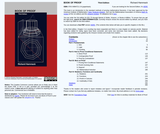Only Sharing Permitted
CC BY-ND
Rating

This book will initiate you into an esoteric world. You will learn and apply the methods of thought that mathematicians use to verify theorems, explore mathematical truth and create new mathematical theories. This will prepare you for advanced mathematics courses, for you will be better able to understand proofs, write your own proofs and think critically and inquisitively about mathematics.

Subject:
Functions
Material Type:
Textbook
Provider:
Virginia Commonwealth University
Author:
Richard Hammack
01/01/2009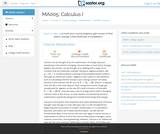Unrestricted Use
CC BY
Rating

This course begins with a review of algebra specifically designed to help and prepare the student for the study of calculus, and continues with discussion of functions, graphs, limits, continuity, and derivatives. The appendix provides a large collection of reference facts, geometry, and trigonometry that will assist in solving calculus problems long after the course is over. Upon successful completion of this course, the student will be able to: calculate or estimate limits of functions given by formulas, graphs, or tables by using properties of limits and LĺÎĺ_ĺĚĺ_hopitalĺÎĺ_ĺĚĺ_s Rule; state whether a function given by a graph or formula is continuous or differentiable at a given point or on a given interval and justify the answer; calculate average and instantaneous rates of change in context, and state the meaning and units of the derivative for functions given graphically; calculate derivatives of polynomial, rational, common transcendental functions, and implicitly defined functions; apply the ideas and techniques of derivatives to solve maximum and minimum problems and related rate problems, and calculate slopes and rates for function given as parametric equations; find extreme values of modeling functions given by formulas or graphs; predict, construct, and interpret the shapes of graphs; solve equations using NewtonĺÎĺ_ĺĚĺ_s Method; find linear approximations to functions using differentials; festate in words the meanings of the solutions to applied problems, attaching the appropriate units to an answer; state which parts of a mathematical statement are assumptions, such as hypotheses, and which parts are conclusions. This free course may be completed online at any time. It has been developed through a partnership with the Washington State Board for Community and Technical Colleges; the Saylor Foundation has modified some WSBCTC materials. (Mathematics 005)

Subject:
Calculus
Functions
Material Type:
Assessment
Full Course
Syllabus
Textbook
Provider:
The Saylor Foundation
10/24/2019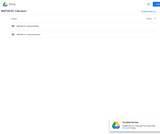Unrestricted Use
CC BY
Rating

This course is an introduction to contemporary calculus and is the first of a three-part sequence. In this course students explore the concepts, applications, and techniques of Calculus - the mathematics of change. Calculus has wide-spread application in science, economics and engineering, and is a foundation college course for further work in these areas. This is a required class for most science and mathematics majors.Login: guest_oclPassword: ocl

Subject:
Calculus
Functions
Material Type:
Full Course
Homework/Assignment
Lecture Notes
Lesson Plan
Syllabus
Provider:
Washington State Board for Community & Technical Colleges
Provider Set:
Open Course Library
10/31/2011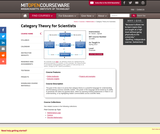Conditional Remix & Share Permitted
CC BY-NC-SA
Rating

The goal of this class is to prove that category theory is a powerful language for understanding and formalizing common scientific models. The power of the language will be tested by its ability to penetrate into taken-for-granted ideas, either by exposing existing weaknesses or flaws in our understanding, or by highlighting hidden commonalities across scientific fields.

Subject:
Functions
Material Type:
Full Course
Provider:
M.I.T.
Provider Set:
M.I.T. OpenCourseWare
Author:
David I. Spivak
01/01/2013Conditional Remix & Share Permitted
CC BY-NC
Rating

This material covers Chapter 3: Functions chapter of the OpenStax College Algebra Text. This module contains an overview of learning objectives mapped to the OTM state standards, worksheets that correspond to chapter sections, interactive Desmos Activities that pair with the chapter, and a list of supplemental videos that correspond to the chapter content.

Subject:
Mathematics
Algebra
Functions
Material Type:
Module
Author:
OER Librarian
05/07/2021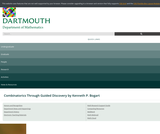Rating

Subject:
Mathematics
Functions
Material Type:
Textbook
Provider:
Dartmouth College
Author:
Kenneth Bogart
01/01/2004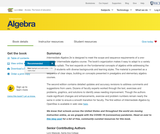Unrestricted Use
CC BY
Rating

The 2nd Edition of Intermediate Algebra, Intermediate Algebra 2e, is designed to meet the scope and sequence requirements of a one-semester intermediate algebra course. The book’s organization makes it easy to adapt to a variety of course syllabi. The text expands on the fundamental concepts of algebra while addressing the needs of students with diverse backgrounds and learning styles. The material is presented as a sequence of clear steps, building on concepts presented in prealgebra and elementary algebra courses.

The second edition contains detailed updates and accuracy revisions to address comments and suggestions from users. Dozens of faculty experts worked through the text, exercises and problems, graphics, and solutions to identify areas needing improvement. Though the authors made significant changes and enhancements, exercise and problem numbers remain nearly the same in order to ensure a smooth transition for faculty.

Subject:
Mathematics
Algebra
Functions
Material Type:
Full Course
Lecture Notes
Textbook
Provider:
Rice University
Provider Set:
OpenStax College
Author:
Andrea Honeycutt Mathis
Lynn Marecek
09/22/2020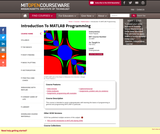Conditional Remix & Share Permitted
CC BY-NC-SA
Rating

This course is intended to assist undergraduates with learning the basics of programming in general and programming MATLAB in particular.

Subject:
Computer Science
Information Science
Functions
Material Type:
Full Course
Provider:
M.I.T.
Provider Set:
M.I.T. OpenCourseWare
Author:
Yossi Farjoun
01/01/2011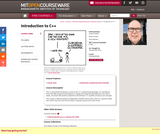Conditional Remix & Share Permitted
CC BY-NC-SA
Rating

This is a fast-paced introductory course to the C++ programming language. It is intended for those with little programming background, though prior programming experience will make it easier, and those with previous experience will still learn C++-specific constructs and concepts. This course is offered during the Independent Activities Period (IAP), which is a special 4-week term at MIT that runs from the first week of January until the end of the month.

Subject:
Computer Science
Information Science
Functions
Material Type:
Full Course
Provider:
M.I.T.
Provider Set:
M.I.T. OpenCourseWare
Author:
Dunietz, Jesse
Kovacs, Geza
Marrero, John
01/01/2010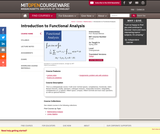Conditional Remix & Share Permitted
CC BY-NC-SA
Rating

" This is a undergraduate course. It will cover normed spaces, completeness, functionals, Hahn-Banach theorem, duality, operators; Lebesgue measure, measurable functions, integrability, completeness of L-p spaces; Hilbert space; compact, Hilbert-Schmidt and trace class operators; as well as spectral theorem."

Subject:
Functions
Material Type:
Full Course
Provider:
M.I.T.
Provider Set:
M.I.T. OpenCourseWare
Author:
Melrose, Richard
01/01/2009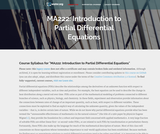Unrestricted Use
CC BY
Rating

Partial differential equations (PDEs) describe the relationships among the derivatives of an unknown function with respect to different independent variables, such as time and position. Experiment and observation provide information about the connections between rates of change of an important quantity, such as heat, with respect to different variables. Upon successful completion of this course, the student will be able to: State the heat, wave, Laplace, and Poisson equations and explain their physical origins; Define harmonic functions; State and justify the maximum principle for harmonic functions; State the mean value property for harmonic functions; Define linear operators and identify linear operations; Identify and classify linear PDEs; Identify homogeneous PDEs and evolution equations; Relate solving homogeneous linear PDEs to finding kernels of linear operators; Define boundary value problem and identify boundary conditions as periodic, Dirichlet, Neumann, or Robin (mixed); Explain physical significance of boundary conditions; Show uniqueness of solutions to the heat, wave, Laplace and Poisson equations with various boundary conditions; Define well-posedness; Define, characterize, and use inner products; Define the space of L2 functions, state its key properties, and identify L2 functions; Define orthogonality and orthonormal basis and show the orthogonality of certain trigonometric functions; Distinguish between pointwise, uniform, and L2 convergence and show convergence of Fourier series; Define Fourier series on [0,pi] and [0,L] and identify sufficient conditions for their convergence and uniqueness; Compute Fourier coefficients and construct Fourier series; Use the method of characteristics to solve linear and nonlinear first-order wave equations; Solve the one-dimensional wave equation using d'Alembert's formula; Use similarity methods to solve PDEs; Solve the heat, wave, Laplace, and Poisson equations using separation of variables and apply boundary conditions; Define the delta function and apply ideas from calculus and Fourier series to generalized functions; Derive Green's representation formula; Use Green's functions to solve the Poisson equation on the unit disk; Define the Fourier transform; Derive basic properties of the Fourier transform of a function, such as its relationship to the Fourier transform of the derivative; Show that the inverse Fourier transform of a product is a convolution; Compute Fourier transforms of functions; Use the Fourier transform to solve the heat and wave equations on unbounded domains. (Mathematics 222)

Subject:
Functions
Material Type:
Full Course
Provider:
The Saylor Foundation
10/24/2019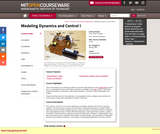Conditional Remix & Share Permitted
CC BY-NC-SA
Rating

First of two-term sequence on modeling, analysis and control of dynamic systems. Mechanical translation, uniaxial rotation, electrical circuits and their coupling via levers, gears and electro-mechanical devices. Analytical and computational solution of linear differential equations and state-determined systems. Laplace transforms, transfer functions. Frequency response, Bode plots. Vibrations, modal analysis. Open- and closed-loop control, instability. Time-domain controller design, introduction to frequency-domain control design techniques. Case studies of engineering applications.

Subject:
Functions
Material Type:
Full Course
Provider:
M.I.T.
Provider Set:
M.I.T. OpenCourseWare
Author:
Dubowsky, Steven
Trumper, David L.
01/01/2005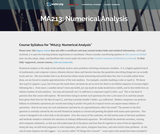Unrestricted Use
CC BY
Rating

Numerical analysis is the study of the methods used to solve problems involving continuous variables. It is a highly applied branch of mathematics and computer science, wherein abstract ideas and theories become the quantities describing things we can actually touch and see. Suggested prerequisites for this course are MA211: Linear Algebra, MA221: Differential Equations, and either MA302/CS101: Introduction to Computer Science, or a background in some programming language. Programming ideas will be illustrated in pseudocode and implemented in the open-source high-level computing environment. Upon successful completion of this course, the student will be able to: show how numbers are represented on the computer, and how errors from this representation affect arithmetic; analyze errors and have an understanding of error estimation; be able to use polynomials in several ways to approximate both functions and data, and to match the type of polynomial approximation to a given type of problem; be able to solve equations in one unknown real variable using iterative methods and to understand how long these methods take to converge to a solution; derive formulas to approximate the derivative of a function at a point, and formulas to compute the definite integral of a function of one or more variables; choose and apply any of several modern methods for solving systems of initial value problems based on properties of the problem. This free course may be completed online at any time. (Mathematics 213)

Subject:
Computer Science
Functions
Material Type:
Full Course
Provider:
The Saylor Foundation
10/24/2019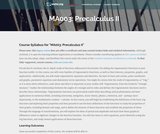Unrestricted Use
CC BY
Rating

This course begins by establishing the definitions of the basic trig functions and exploring their properties, and then proceeds to use the basic definitions of the functions to study the properties of their graphs, including domain and range, and to define the inverses of these functions and establish the their properties. Through the language of transformation, the student will explore the ideas of period and amplitude and learn how these graphical differences relate to algebraic changes in the function formulas. The student will also learn to solve equations, prove identities using the trig functions, and study several applications of these functions. Upon successful completion of this course, the student will be able to: measure angles in degrees and radians, and relate them to arc length; solve problems involving right triangles and unit circles using the definitions of the trigonometric functions; solve problems involving non-right triangles; relate the equation of a trigonometric function to its graph; solve trigonometric equations using inverse trig functions; prove trigonometric identities; solve trig equations involving identities; relate coordinates and equations in Polar form to coordinates and equations in Cartesian form; perform operations with vectors and use them to solve problems; relate equations and graphs in Parametric form to equations and graphs in Cartesian form; link graphical, numeric, and symbolic approaches when interpreting situations and analyzing problems; write clear, correct, and complete solutions to mathematical problems using proper mathematical notation and appropriate language; communicate the difference between an exact and an approximate solution and determine which is more appropriate for a given problem. This free course may be completed online at any time. It has been developed through a partnership with the Washington State Board for Community and Technical Colleges; the Saylor Foundation has modified some WSBCTC materials. (Mathematics 003)

Subject:
Functions
Material Type:
Full Course
Provider:
The Saylor Foundation
10/24/2019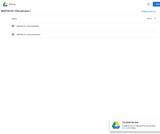Unrestricted Use
CC BY
Rating

This course will cover families of functions, their properties, graphs and applications. These functions include: polynomial, rational, exponential, logarithmic functions and combinations of these. We will solve related equations and inequalities and conduct data analysis, introductory mathematical modeling and develop competency with a graphing calculator.Login: guest_oclPassword: ocl

Subject:
Functions
Material Type:
Full Course
Homework/Assignment
Lecture Notes
Lesson Plan
Syllabus
Provider:
Washington State Board for Community & Technical Colleges
Provider Set:
Open Course Library
10/31/2011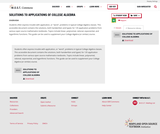Conditional Remix & Share Permitted
CC BY-NC-SA
Rating

Students often express trouble with application, or "word", problems in typical College Algebra classes. This accessible document contains the solutions, both handwritten and typed, for 135 application problems from various open source mathematics textbooks. Topics include linear, polynomial, rational, exponential, and logarithmic functions. This guide can be used to supplement your College Algebra (or similar) course.

Subject:
Algebra
Functions
Material Type:
Author:
Jesse Kiefner
DANIELLE TRUSZKOWSKI
06/10/2020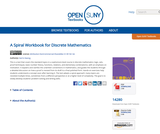Conditional Remix & Share Permitted
CC BY-NC-SA
Rating

This is a text that covers the standard topics in a sophomore-level course in discrete mathematics: logic, sets, proof techniques, basic number theory, functions, relations, and elementary combinatorics, with an emphasis on motivation. It explains and clarifies the unwritten conventions in mathematics, and guides the students through a detailed discussion on how a proof is revised from its draft to a final polished form. Hands-on exercises help students understand a concept soon after learning it. The text adopts a spiral approach: many topics are revisited multiple times, sometimes from a different perspective or at a higher level of complexity. The goal is to slowly develop students’ problem-solving and writing skills.

Subject:
Mathematics
Functions
Material Type:
Textbook
Provider:
State University of New York
Provider Set:
OpenSUNY Textbooks
Author:
Harris Kwong, SUNY Fredonia